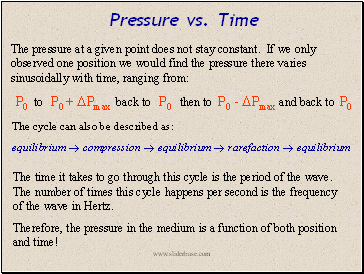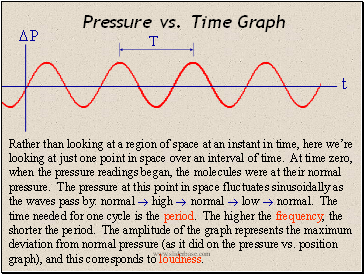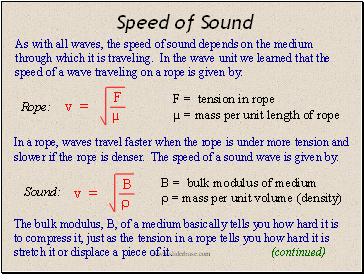# SoundPage 2

#### WATCH ALL SLIDES

B

Pressure vs. Position Graph

 P

x

A

C

A:  P = 0; P = P0

B:  P > 0; P = Pmax

C:  P < 0; P = Pmin

animation

Slide 6## Pressure vs. Time

The pressure at a given point does not stay constant. If we only observed one position we would find the pressure there varies sinusoidally with time, ranging from:

P0 to P0 +  Pmax back to P0 then to P0 -  Pmax and back to P0

The time it takes to go through this cycle is the period of the wave. The number of times this cycle happens per second is the frequency of the wave in Hertz.

Therefore, the pressure in the medium is a function of both position and time!

The cycle can also be described as:

equilibrium  compression  equilibrium  rarefaction  equilibrium

Slide 7## Pressure vs. Time Graph

 P

T

t

Rather than looking at a region of space at an instant in time, here we’re looking at just one point in space over an interval of time. At time zero, when the pressure readings began, the molecules were at their normal pressure. The pressure at this point in space fluctuates sinusoidally as the waves pass by: normal  high  normal  low  normal. The time needed for one cycle is the period. The higher the frequency, the shorter the period. The amplitude of the graph represents the maximum deviation from normal pressure (as it did on the pressure vs. position graph), and this corresponds to loudness.

Slide 8## Comparison of Pressure Graphs

Pressure vs. Position: The graph is for a snapshot in time and displays pressure variation for over an interval of space. The distance between peaks on the graph is the wavelength of the wave.

Pressure vs. Time: The graph displays pressure variation over an interval of time for only one point in space. The distance between peaks on the graph is the period of the wave. The reciprocal of the period is the frequency.

Both Graphs: Sound waves are longitudinal even though these graphs look like transverse waves. Nothing in a sound wave is actually waving in the shape of these graphs! The amplitude of either graph corresponds to the loudness of the sound. The absolute pressure matters not. For loudness, all that matters is how much the pressure deviates from its norm, which doesn’t have to be much. In real life the amplitude would diminish as the sound waves spread out.

Slide 9## Speed of Sound

As with all waves, the speed of sound depends on the medium through which it is traveling. In the wave unit we learned that the speed of a wave traveling on a rope is given by:

Go to page:
1  2  3  4  5  6  7  8  9  10  11  12  13